## Thursday, May 23, 2019

 table div table+table+table div table{width:100%;padding:0}table div table+table+table div table img{width:96.23%;padding:0;float:none}table div table+table+table div table td{width:100%;padding:0 1.88% 18px}/* styles */# Sunday's Service - May 26, 2019

 /* styles */ We welcome back Adam Shank to lead us in Worship this week. The featured Scripture verses are: 1 Peter 1:13-21 for Adam's message: Holy Father, Holy Children. We will celebrate the Sacrament of Communion.
 /* styles */ ~~~

# CG NEWS & EVENTS## Bible Studies

Sunday Morning Class: Romans, 9:30a.m.
Wednesday Morning Ladies Study: Hosea, 9:30am at Jen Shannon's

You are welcome to join in studies at any time!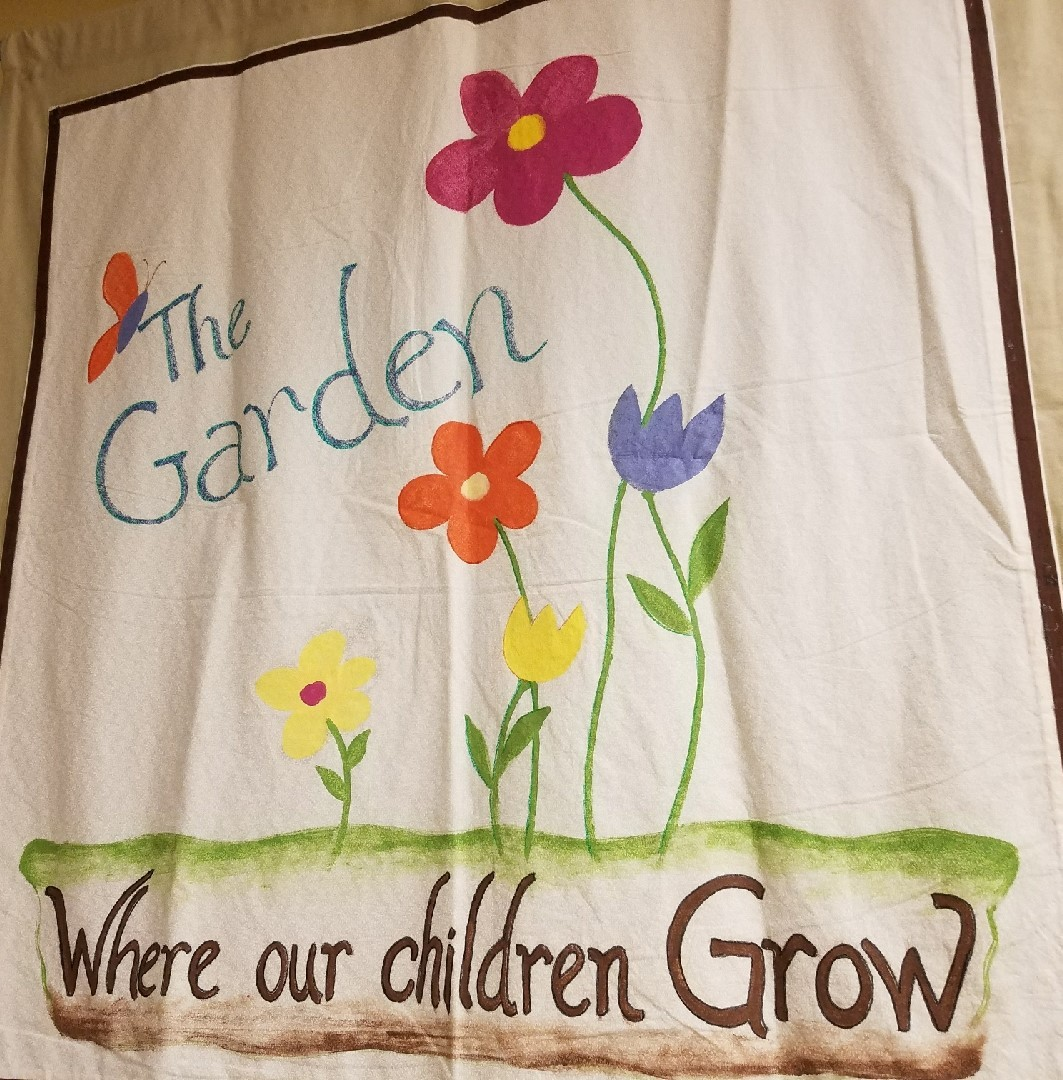## Garden & Nursery News

The Garden is on Summer Break until September 8th. Activity pages will be on the table by the Sanctuary doors. Children 3 & younger are invited to the Nursery for Bible story time.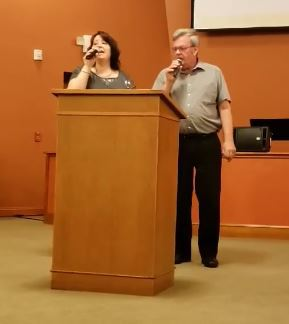## Tried & True

Sunday's musical service was full of joy and appreciated by everyone. Thank you to Denise and Lynn for sharing your gifts with us. We certainly want you back before another five years passes!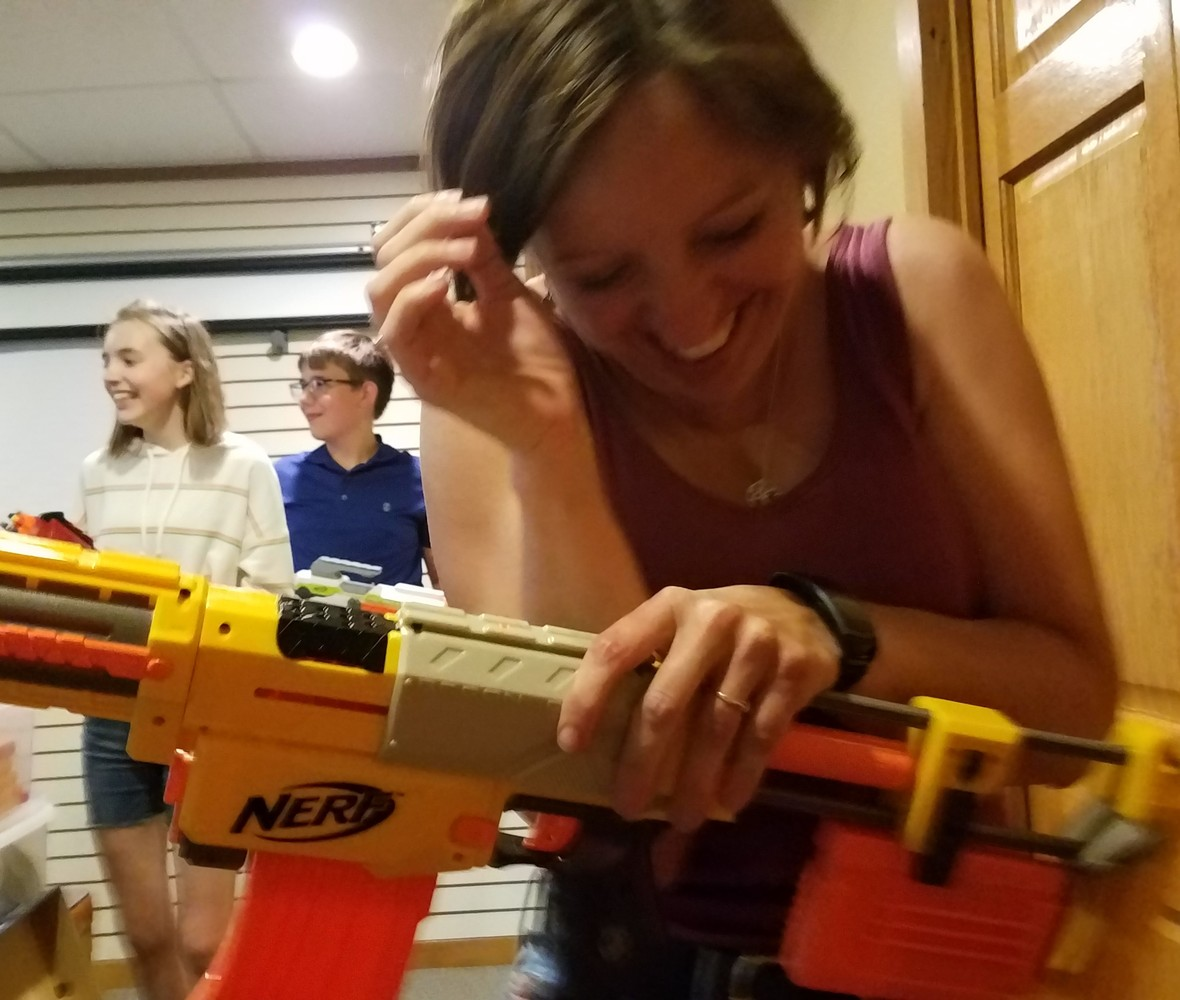## Youth Group

We had a fantastic and fun final meeting! We discussed
Proverbs 16:9 and God's awesome plans for our future,
enjoyed some Italian ice, and finished out the school year with
a rollicking Nerf Battle. Thanks, everyone, for a great year!
Summer plans will be scheduled soon.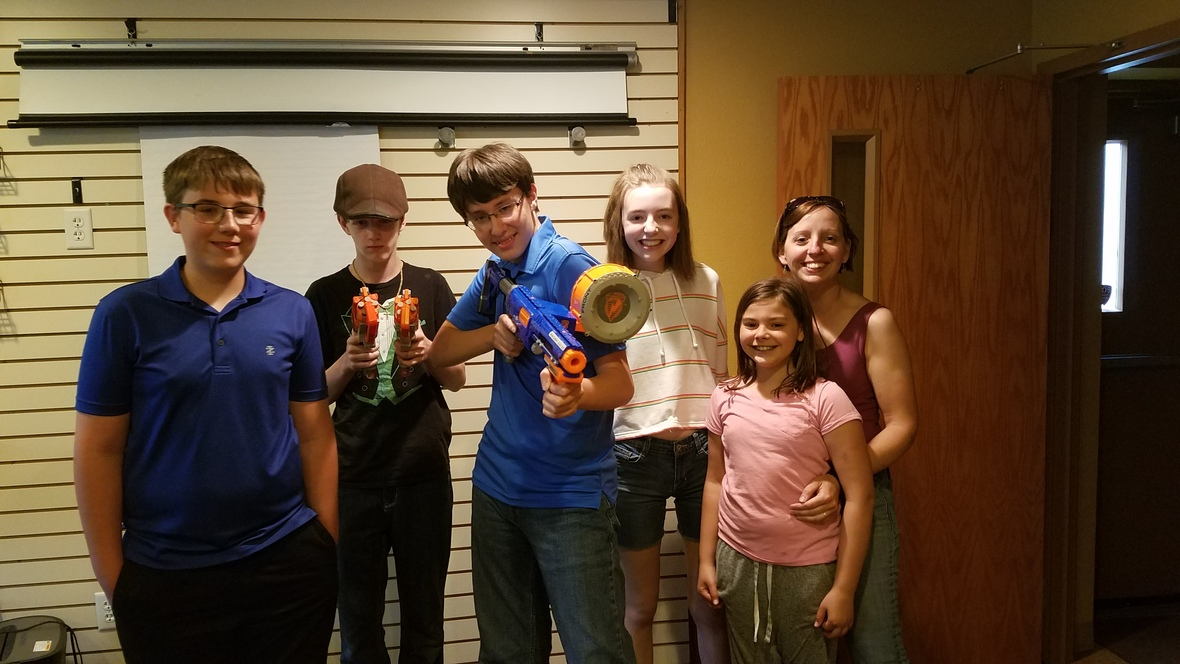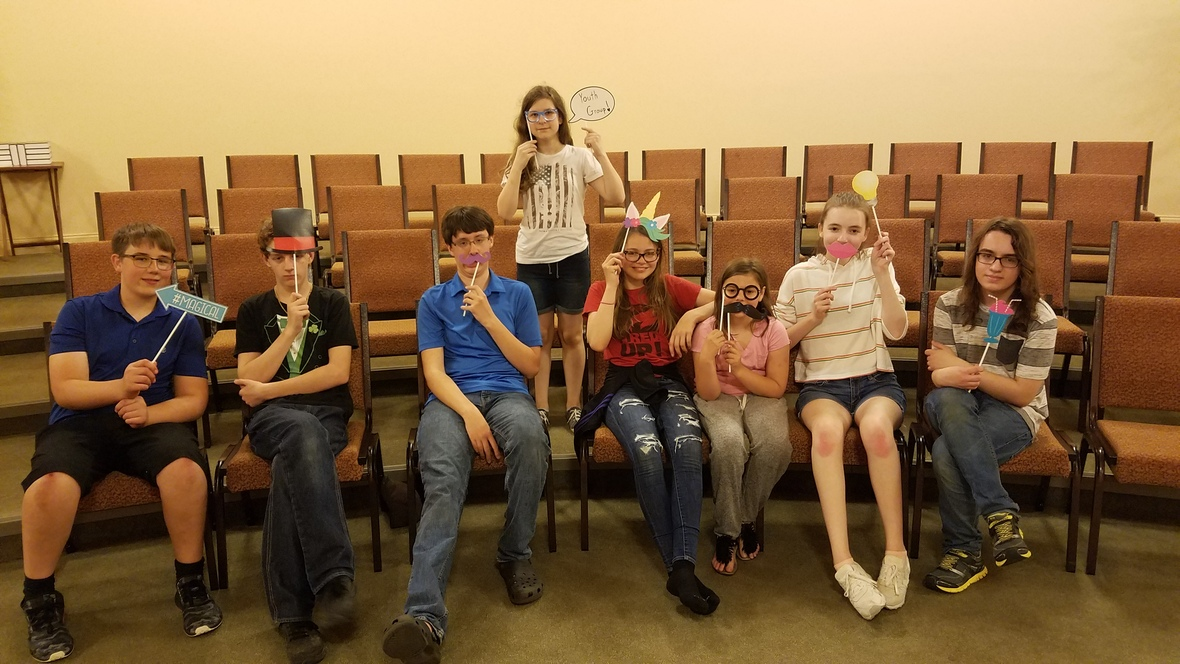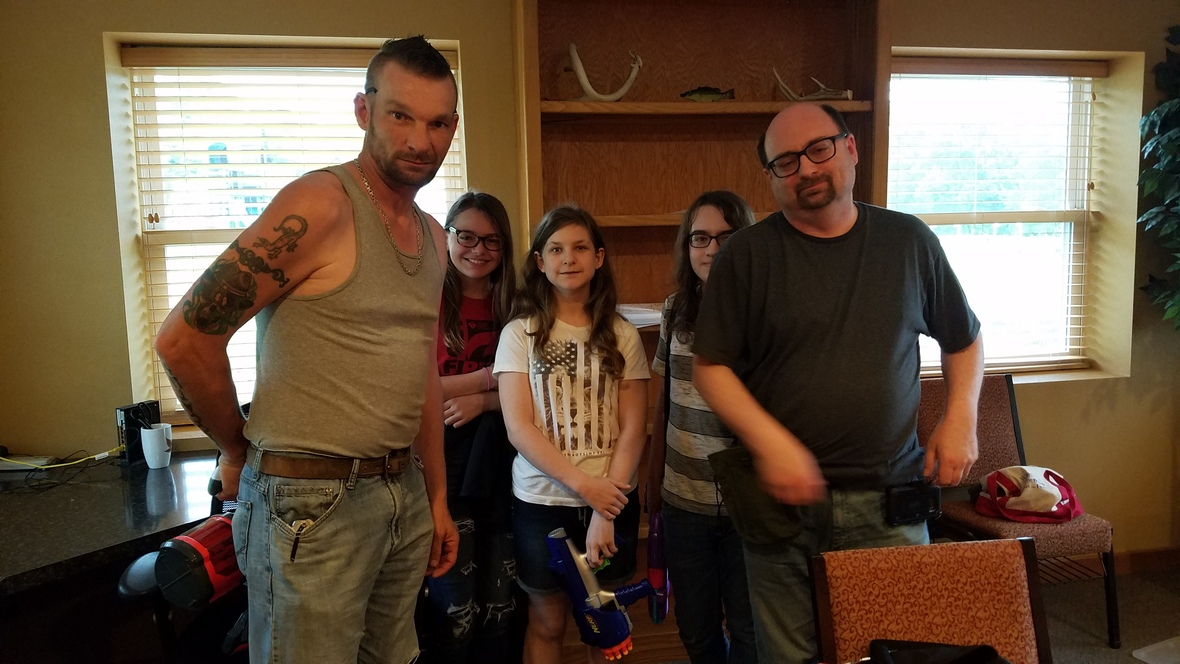table div table+table+table+table+table+table+table+table+table+table+table+table+table+table div table{width:100%;padding:0}table div table+table+table+table+table+table+table+table+table+table+table+table+table+table div table img{width:96.23%;padding:0;float:none}table div table+table+table+table+table+table+table+table+table+table+table+table+table+table div table td{width:100%;padding:0 1.88% 18px}/* styles */# UPCOMING AT CG## June Speakers

June 2 - Phil Bartholomew
June 9 - Phil Bartholomew
June 16 - John Muckridge
June 30 - Adam Shank - Communion Sunday

 table div table+table+table+table+table+table+table+table+table+table+table+table+table+table+table+table+table div table{width:100%;padding:0}table div table+table+table+table+table+table+table+table+table+table+table+table+table+table+table+table+table div table img{width:96.23%;padding:0;float:none}table div table+table+table+table+table+table+table+table+table+table+table+table+table+table+table+table+table div table td{width:100%;padding:0 1.88% 18px}/* styles *//* styles */ www.goodnessgrows4all.org Our Mission Statement: To cultivate personal, social, and community growth through gardening, education, and opportunity for people of all abilities. ~~~

# NEWS & EVENTS## Fern Sale

Boston ferns available! Limited supply, \$18 + tax. Buy some beauty for your home, porch, or yard while raising money to support the work of Goodness Grows. Check them out in the greenhouse on Sunday or stop by Monday-Friday from 8:30am-2:30pm, though you may want to call to make sure we are available and not on a field trip. A great big thank you to everyone who has already purchased a fern!

## NEXT Weekend, Come See Us At...

 table.module-22{width:0%;padding:0}table div table+table+table+table+table+table+table+table+table+table+table+table+table+table+table+table+table+table+table+table+table+table+table div table{width:0%;float:none;margin-left:auto;margin-right:auto;padding:0}table div table+table+table+table+table+table+table+table+table+table+table+table+table+table+table+table+table+table+table+table+table+table+table div table a{border:0 none;text-decoration:none}table div table+table+table+table+table+table+table+table+table+table+table+table+table+table+table+table+table+table+table+table+table+table+table div table img{width:100%!important;border:0 none;text-decoration:none}table div table+table+table+table+table+table+table+table+table+table+table+table+table+table+table+table+table+table+table+table+table+table+table div table td{width:100%;padding:0}/* styles */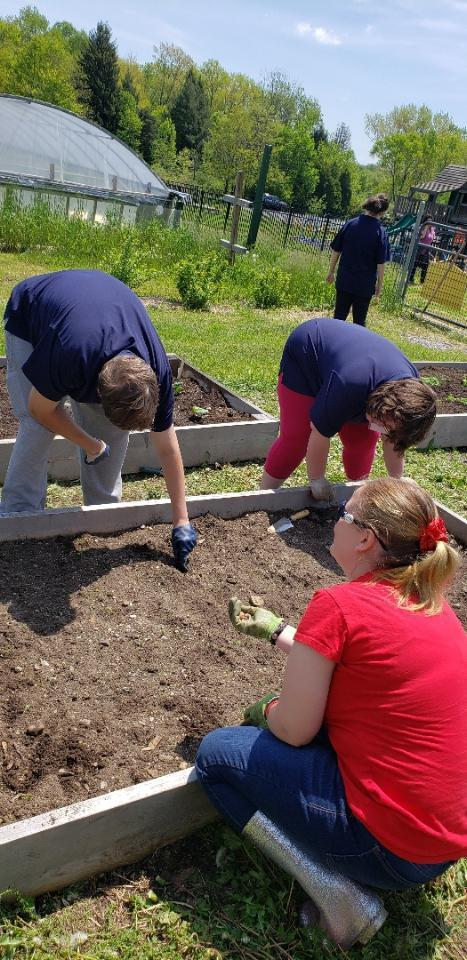## Summer is Here at GG!

This week marks the beginning of our summer season. Our final classroom visit is today, with Salem. After this we won't see our school friends until fall. A big thank you to all the hard working students, teachers, and staff who spent their spring semester with us! You guys are all awesome! Have a great summer and we look forward to your return in September.

With the ending of the school year, planting and other farm chores jump into high gear. This means all hands on deck as our summer workers return. We were very excited to welcome back long time GG employee Trevor Wilson yesterday! He got right to work helping clean out the warehouse. Now we just wait impatiently for the rest of our crew to start.

Monday is the final day to register for this year's CSA program. If you want high quality, local fruits and veggies grown right here, let us know before it is too late!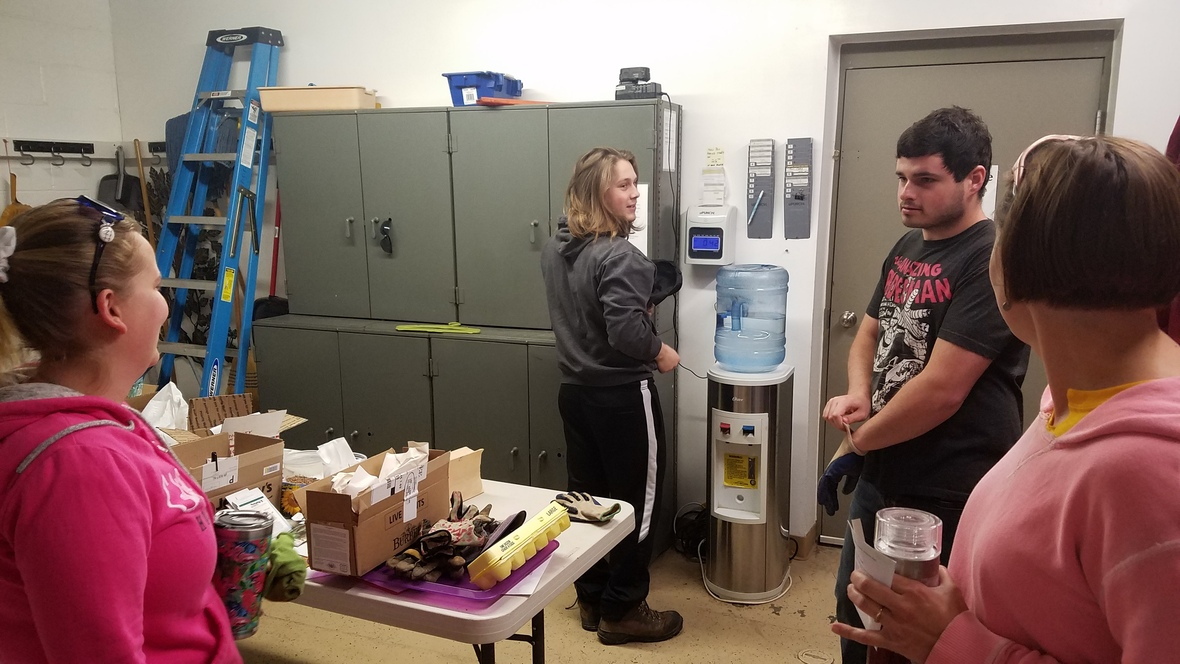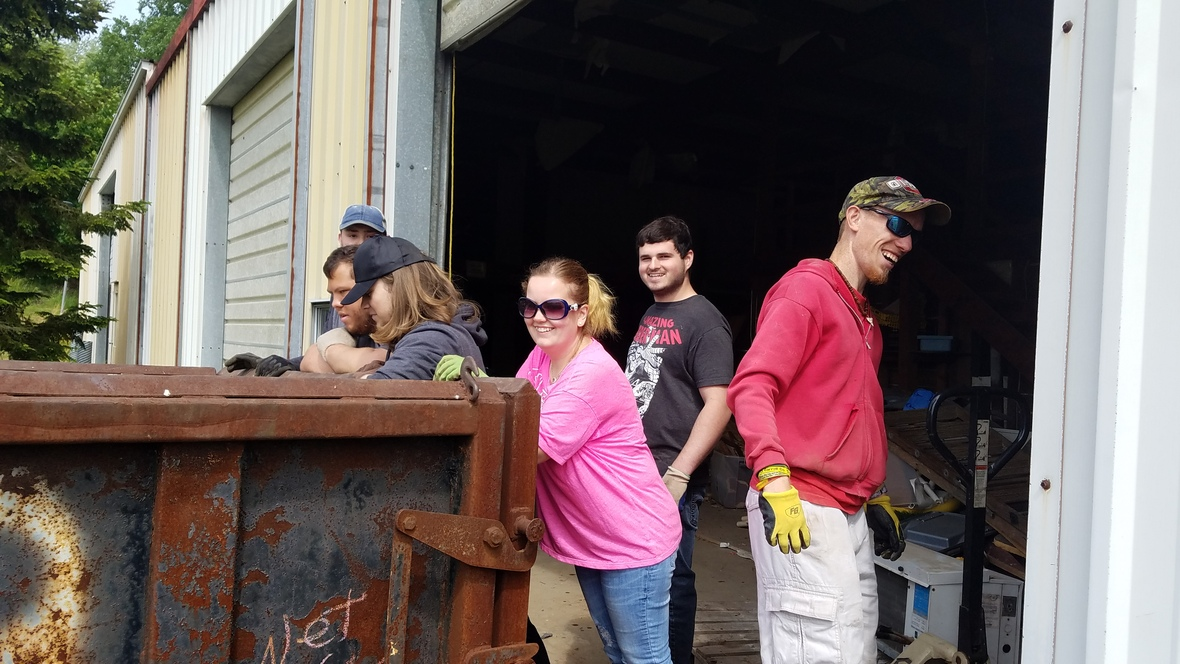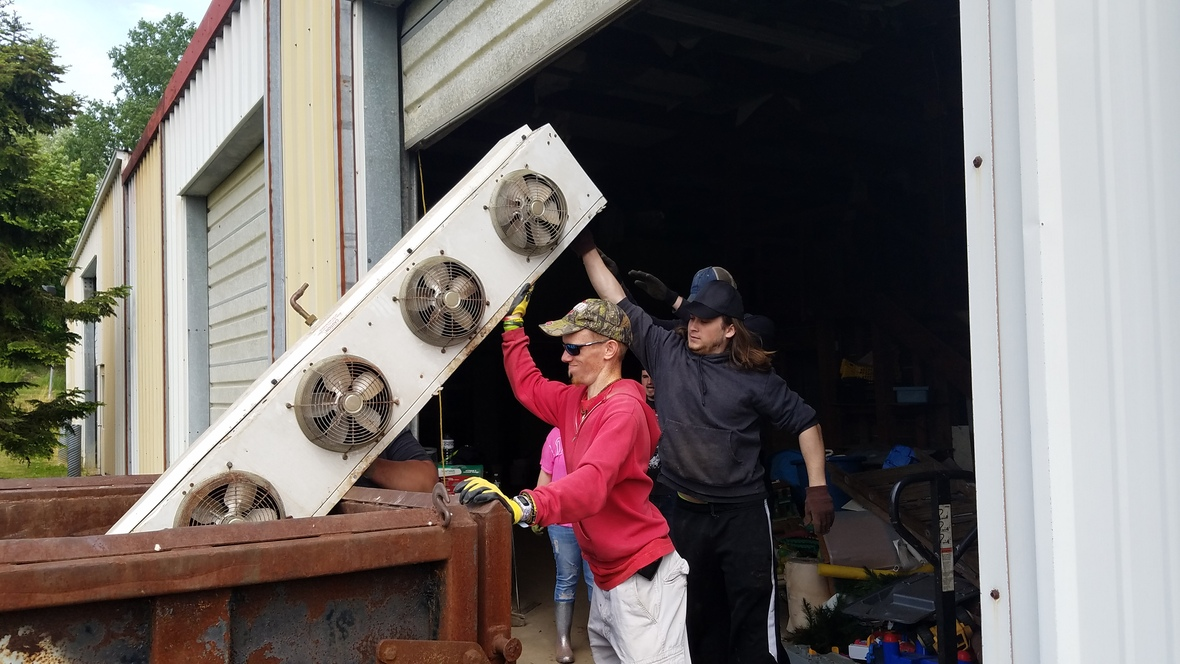table div table+table+table+table+table+table+table+table+table+table+table+table+table+table+table+table+table+table+table+table+table+table+table+table+table+table div table{width:100%;padding:0}table div table+table+table+table+table+table+table+table+table+table+table+table+table+table+table+table+table+table+table+table+table+table+table+table+table+table div table img{width:96.23%;padding:0;float:none}table div table+table+table+table+table+table+table+table+table+table+table+table+table+table+table+table+table+table+table+table+table+table+table+table+table+table div table td{width:100%;padding:0 1.88% 18px}/* styles */# OUTREACH and MISSION OPPORTUNITIES## Coat & Clothing Drive

Ongoing Outreach/Mission Opportunity
Drop items off in the collection barrel or take them up to the Green Room, anytime throughout the year! Let your friends and family know that we accept donations year-round. We are happy to take coats, clothes, shoes, purses and bags, jewelry, hats, gloves and mittens, scarves, and even small household items. Anything that is clean and in good repair that you think someone else might be able to use!

Date for Giveaway Set ~ Saturday, October 12th, 10am-1pm!

 /* styles */ ~~
 /* styles */ For I was hungry and you gave me food; I was thirsty and you gave me a drink; I was a stranger and you took me in; I was naked and you clothed me; I was sick and you visited me. I was in prison and you came to me. .....When you did it to one of the least of these my brothers and sisters, you were doing it to me. Matthew 25: 35-36, 40 ~ What can YOU do?
 table div table+table+table+table+table+table+table+table+table+table+table+table+table+table+table+table+table+table+table+table+table+table+table+table+table+table+table+table+table+table+table div table{width:100%;padding:0}table div table+table+table+table+table+table+table+table+table+table+table+table+table+table+table+table+table+table+table+table+table+table+table+table+table+table+table+table+table+table+table div table img{width:96.23%;padding:0;float:none}table div table+table+table+table+table+table+table+table+table+table+table+table+table+table+table+table+table+table+table+table+table+table+table+table+table+table+table+table+table+table+table div table td{width:100%;padding:0 1.88% 18px}/* styles */# LOCAL COMMUNITY NEWS/EVENTS

 /* styles */ If you know of any interesting news or events, please let us know, so that we can pass it on to others!
 table.module-33{width:0%;padding:0}table div table+table+table+table+table+table+table+table+table+table+table+table+table+table+table+table+table+table+table+table+table+table+table+table+table+table+table+table+table+table+table+table+table+table div table{width:0%;float:none;margin-left:auto;margin-right:auto;padding:0}table div table+table+table+table+table+table+table+table+table+table+table+table+table+table+table+table+table+table+table+table+table+table+table+table+table+table+table+table+table+table+table+table+table+table div table a{border:0 none;text-decoration:none}table div table+table+table+table+table+table+table+table+table+table+table+table+table+table+table+table+table+table+table+table+table+table+table+table+table+table+table+table+table+table+table+table+table+table div table img{width:100%!important;border:0 none;text-decoration:none}table div table+table+table+table+table+table+table+table+table+table+table+table+table+table+table+table+table+table+table+table+table+table+table+table+table+table+table+table+table+table+table+table+table+table div table td{width:100%;padding:0}/* styles */## Beaver Township Memorial Day Parade & Ceremony

Monday, May 27 between 11am and 1pm in the center of
North Lima.

 table.module-35{width:0%;padding:0}table div table+table+table+table+table+table+table+table+table+table+table+table+table+table+table+table+table+table+table+table+table+table+table+table+table+table+table+table+table+table+table+table+table+table+table+table div table{width:0%;float:none;margin-left:auto;margin-right:auto;padding:0}table div table+table+table+table+table+table+table+table+table+table+table+table+table+table+table+table+table+table+table+table+table+table+table+table+table+table+table+table+table+table+table+table+table+table+table+table div table a{border:0 none;text-decoration:none}table div table+table+table+table+table+table+table+table+table+table+table+table+table+table+table+table+table+table+table+table+table+table+table+table+table+table+table+table+table+table+table+table+table+table+table+table div table img{width:100%!important;border:0 none;text-decoration:none}table div table+table+table+table+table+table+table+table+table+table+table+table+table+table+table+table+table+table+table+table+table+table+table+table+table+table+table+table+table+table+table+table+table+table+table+table div table td{width:100%;padding:0}/* styles */

## Mill Creek Park Naturalist Series Lecture: Cicadas

May 28, 6-7:30pm at the MetroParks Farm
Learn all about the 17-year cicada that is emerging in the Mahoning Valley. What does it do? Why is it in the ground for 17 years? Are they a problem for pets? Trees? Shrubs? Ashley will share an energetic presentation about the life cycle and wonder of this phenomenal insect. Registration includes program and handouts. Call OSU Extension at 330.533.5538 or Fellows Riverside Gardens at 330.740.7116 to register. Free.

The Naturalist Series is a collaboration between the OSU Extension – Mahoning County Ohio Certified Volunteer Naturalist program and Mill Creek MetroParks. Coyotes, the next program in this series, is on June 20.

## Crown Theater Events at Main Street Theater

Summer Drama Camp Into The Woods for 7th-12th grade starts June 3rd at 9am.
Into The Woods presented by the Jr/Sr High Summer Drama Camp opens June 22nd

My Fair Lady opens June 7th.

Information, registration, and tickets all available HERE.

 table div table+table+table+table+table+table+table+table+table+table+table+table+table+table+table+table+table+table+table+table+table+table+table+table+table+table+table+table+table+table+table+table+table+table+table+table+table+table+table div table{width:100%;padding:0}table div table+table+table+table+table+table+table+table+table+table+table+table+table+table+table+table+table+table+table+table+table+table+table+table+table+table+table+table+table+table+table+table+table+table+table+table+table+table+table div table img{width:96.23%;padding:0;float:none}table div table+table+table+table+table+table+table+table+table+table+table+table+table+table+table+table+table+table+table+table+table+table+table+table+table+table+table+table+table+table+table+table+table+table+table+table+table+table+table div table td{width:100%;padding:0 1.88% 18px}/* styles */# CELEBRATE!

Today! - Heidi Landis
May 24 - National Scavenger Hunt Day
May 27 - Memorial Day ~ In remembrance of those who died while serving in the military.
May 29 - Learn About Composting Day ~ An easy way to Reduce, Reuse, Recycle!

 table.module-40{width:0%;padding:0}table div table+table+table+table+table+table+table+table+table+table+table+table+table+table+table+table+table+table+table+table+table+table+table+table+table+table+table+table+table+table+table+table+table+table+table+table+table+table+table+table+table div table{width:0%;float:none;margin-left:auto;margin-right:auto;padding:0}table div table+table+table+table+table+table+table+table+table+table+table+table+table+table+table+table+table+table+table+table+table+table+table+table+table+table+table+table+table+table+table+table+table+table+table+table+table+table+table+table+table div table a{border:0 none;text-decoration:none}table div table+table+table+table+table+table+table+table+table+table+table+table+table+table+table+table+table+table+table+table+table+table+table+table+table+table+table+table+table+table+table+table+table+table+table+table+table+table+table+table+table div table img{width:100%!important;border:0 none;text-decoration:none}table div table+table+table+table+table+table+table+table+table+table+table+table+table+table+table+table+table+table+table+table+table+table+table+table+table+table+table+table+table+table+table+table+table+table+table+table+table+table+table+table+table div table td{width:100%;padding:0}/* styles */

# ON THE CG CALENDAR

May 23-29. . .
Today, 10am - GG/Salem Class
Sunday, 9:30am - Bible Study
Sunday, 11am - Worship
Monday - Office & GG Closed, CSA Deadline
Wednesday, 9:30am - Ladies Bible Study/* styles */ Seeking to reflect the unconditional love of God, our mission is to help people connect - to God, to one another, and to God's purpose for the world.
 table div table+table+table+table+table+table+table+table+table+table+table+table+table+table+table+table+table+table+table+table+table+table+table+table+table+table+table+table+table+table+table+table+table+table+table+table+table+table+table+table+table+table+table+table+table div table{width:100%;padding:0}table div table+table+table+table+table+table+table+table+table+table+table+table+table+table+table+table+table+table+table+table+table+table+table+table+table+table+table+table+table+table+table+table+table+table+table+table+table+table+table+table+table+table+table+table+table div table img{width:96.23%;padding:0;float:none}table div table+table+table+table+table+table+table+table+table+table+table+table+table+table+table+table+table+table+table+table+table+table+table+table+table+table+table+table+table+table+table+table+table+table+table+table+table+table+table+table+table+table+table+table+table div table td{width:100%;padding:0 1.88% 18px}/* styles *//* styles */ Come grow with us! Service Time: Sunday @ 11:00am Common Ground Church Community 2310 West South Range Road North Lima, Ohio 44452 ~~~~~~~~~~~~~~~~~~~~~~~~~~~~~~~~~~~~~~~~~~~~ For more information, call 330-549-9408 or contact us by email at commonground.secretary@gmail.com.
 /* styles */